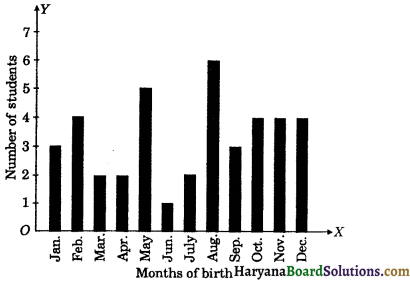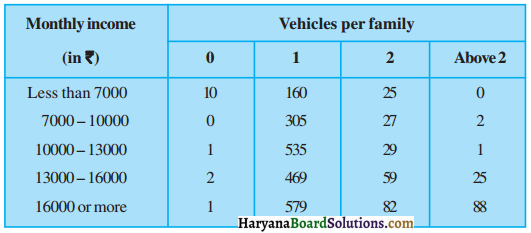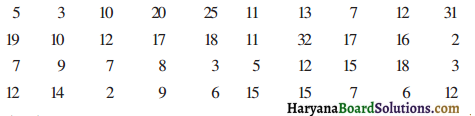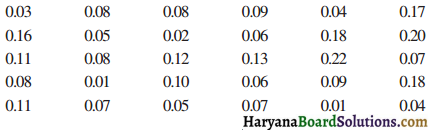# HBSE 9th Class Maths Solutions Chapter 15 Probability Ex 15.1

Haryana State Board HBSE 9th Class Maths Solutions Chapter 15 Probability Ex 15.1 Textbook Exercise Questions and Answers.

## Haryana Board 9th Class Maths Solutions Chapter 15 Probability Exercise 15.1

Question 1.
In a cricket match, a batswoman hits a boundary 6 times out of 30 balls she plays. Find the probability that she did not hit a boundary.
Solution:
Number of balls thrown = 30
∴ Total number of possible outcomes = 30
Number of times, the boundary is hit by the ball = 6
Number of times, the boundary is not hit by the ball= 30 – 6 = 24
∴ Number of favourable outcomes = 24
Let E be event that the boundary is not hit by the ball
∴ P(E) = $$\frac{\text { Number of favourable outcomes }}{\text { Total number of possible outcomes }}$$
= $$\frac{24}{30}$$ = $$\frac{4}{5}$$
Hence, P(E) = $$\frac{4}{5}$$

Question 2.
1500 families with 2 children were selected randomly, and the following data were recorded :

 Number of girls in a family 2 1 0 Number of families 475 814 211

Compute the probability of a family, chosen at random, having :
(i) 2 girls
(ii) 1 girl
(iii) No girl.
Also check whether the sum of these probabilities is 1.
Solution:
Total number of families = 1500
∴ Total number of possible outcomes = 1500
(i) Number of families having 2 girls = 475
∴ Number of favourable outcomes = 475
Let E1 be event that the selected family has 2 girls, then
P(E1) = $$\frac{\text { Number of favourable outcomes }}{\text { Total number of possible outcomes }}$$
= $$\frac{475}{1500}$$ = $$\frac{19}{60}$$

(ii) Number of families having 1 girl = 814
∴ Number of favourable outcomes = 814
Let E2 be the event that the selected family has 1 girl, then
P(E2) = $$\frac{\text { Number of favourable outcomes }}{\text { Total number of possible outcomes }}$$
= $$\frac{814}{1500}$$ = $$\frac{407}{750}$$

(iii) Number of families having no girl = 211
∴ Number of favourable outcomes = 211
Let E3 be the event that the selected family has no girl; then
P(E3) = $$\frac{\text { Number of favourable outcomes }}{\text { Total number of possible outcomes }}$$
= $$\frac{211}{1500}$$

(iv) Sum of the probabilities
= $$\frac{19}{60}+\frac{407}{750}+\frac{211}{1500}$$
= $$\frac{475+814+211}{1500}$$
= $$\frac{1500}{1500}$$ = 1
Hence, (i) P(E1) = $$\frac{19}{60}$$, (ii) P(E2) = $$\frac{407}{750}$$ (iii) P(E3) = $$\frac{211}{1500}$$ (iv) Sum of the probabilities = 1.Question 3.
In a particular section of class IX, 40 students were asked about the month of their birth and the following graph was prepared for the data so obtained :Find the probability that a student of the class was born in August.
Solution :
Total number of students = 40
∴ Total number of possible outcomes = 40
Number of students was born in August = 6
∴ Number of favourable outcomes = 6
Let E be the event that a student of the class was born in August, then
P(E) = $$\frac{\text { Number of favourable outcomes }}{\text { Total number of possible outcomes }}$$
= $$\frac{6}{40}$$ = $$\frac{3}{20}$$
Hence, P(E) = $$\frac{3}{20}$$

Question 4.
Three coins are tossed simultaneously 200 times with the following frequencies of different outcomes :

If the three coins are simultaneously tossed again, compute the probability of 2 heads coming up.
Solution :
Total number of tosses of three coins simultaneously = 200
∴ Total number of possible outcomes = 200
Number of outcomes of 2 heads = 72
∴ Number of favourable outcomes = 72
Let E be the event of 2 heads coming up, then
P(E) = $$\frac{\text { Number of favourable outcomes }}{\text { Total number of possible outcomes }}$$
= $$\frac{72}{200}$$ = $$\frac{9}{25}$$
Hence, P(E) = $$\frac{9}{25}$$Question 5.
An organisation selected 2400 families at random and surveyed them to determine their income level and the number of vehicles in a family. The information gathered is listed in the table below :Suppose a family is chosen. Find the probability that the family chosen is :
(i) earning Rs. 10000 – 13000 per month and owning exactly 2 vehicles.
(ii) earning Rs. 16000 or more per month and owning exactly 1 vehicle.
(iii) earning less than Rs. 7000 per month and does not own any vehicle.
(iv) earning Rs. 13000 – 16000 per month and owning more than 2 vehicles.
(v) owning not more than 1 vehicle.
Solution:
The total number of families = 2400
∴ Total number of possible outcomes= 2400
(i) Number of families earning Rs. 10000 – 13000 per month and owning exactly 2 vehicles = 29
∴ Number of favourable outcomes = 29
Let E1 be the event that a family’s earning 10000 – 13000 per month and owning exactly 2 vehicles, then
P(E1) = $$\frac{\text { Number of favourable outcomes }}{\text { Total number of possible outcomes }}$$
= $$\frac{29}{2400}$$

(ii) Number of families earning Rs. 16000 or more per month and owning exactly 1 vehicle = 579
∴ Number of favourable outcomes = 579
Let E2 be the event that a family’s earning Rs. 16000 or more per month and owning exactly 1 vehicle, then
P(E2) = $$\frac{\text { Number of favourable outcomes }}{\text { Total number of possible outcomes }}$$
= $$\frac{579}{2400}$$

(iii) Number of families earning less than Rs. 7000 per month and does not own any vehicle = 10
∴ Number of favourable outcomes = 10
Let E3 be the event that a family’s earning less than Rs. 7000 per month and does not own any vehicle, then
P(E3) = $$\frac{\text { Number of favourable outcomes }}{\text { Total number of possible outcomes }}$$
= $$\frac{10}{2400}$$ = $$\frac{1}{240}$$

(iv) Number of families earning Rs. 13000 – 16000 per month and owning more than 2 vehicles = 25
∴ Number of favourable outcomes = 25
Let E4 be the event that a family’s earning Rs. 13000 – 16000 per month and owning more than 2 vehicles, then
P(E4) = $$\frac{\text { Number of favourable outcomes }}{\text { Total number of possible outcomes }}$$
= $$\frac{25}{2400}$$ = $$\frac{1}{96}$$

(v) Number of families owning not more than 1 vehicle = 10 + 0 + 1 + 2 + 1 + 160 + 305 + 535 + 469 + 579
= 2062
∴ Number of favourable outcomes= 2062
Let E5 be the event that a family owning not more than 1 vehicle, then
P(E5) = $$\frac{\text { Number of favourable outcomes }}{\text { Total number of possible outcomes }}$$
= $$\frac{2062}{2400}$$ = $$\frac{1031}{1200}$$
Hence,
(i) P(E1) = $$\frac{29}{2400}$$;
(ii) P(E2) = $$\frac{579}{2400}$$
(i) P(E3) = $$\frac{1}{240}$$
(iv) P(E4) = $$\frac{1}{96}$$
(v) P(E5) = $$\frac{1031}{1200}$$

Question 6.
A teacher wanted to analyse the performance of two sections of students in a mathematics test of 100 marks. A data of their performances is given below in the table :

 Marks Number of students 0 – 20 7 20 – 30 10 30 – 40 10 40 – 50 20 50 – 60 20 60 – 70 15 70 – above 8 Total 90

(i) Find the probability that a student obtained less than 20% marks in the mathematics test.
(ii) Find the probability that a student obtained marks 60 or above.
Solution:
Total number of students = 90
∴ Total number of possible outcomes = 90
(i) Number of students who obtained less than 20% marks in the test = 7
∴ Number of favourable outcomes = 7
Let E1 be the event of a student obtaining less than 20% marks in the test.
P(E1) = $$\frac{\text { Number of favourable outcomes }}{\text { Total number of possible outcomes }}$$
= $$\frac{7}{90}$$

(ii) Number of students who obtained marks 60 or above in the test = 15 + 8 = 23
∴ Number of favourable outcomes = 23
Let E2 be the event of a student obtaining 60 or above marks in the test.
P(E2) = $$\frac{\text { Number of favourable outcomes }}{\text { Total number of possible outcomes }}$$
= $$\frac{23}{90}$$
Hence, (i) P(E1) = $$\frac{7}{90}$$,
(ii) P(E2) = $$\frac{23}{90}$$Question 7.
To know the opinion of the students about the subject statistics, a survey of 200 students was conducted. The data is recorded in the following table :

 Opinion Number of students like 135 dislike 65

Find the probability that a student chosen at random :
(i) likes statistics,
(ii) does not like it.
Solution:
Total number of students = 200
∴ Total number of possible outcomes = 200
(i) Number of students who likes statistics = 135
∴ Number of favourable outcomes = 135
Let E1 be the event of a student likes statistics, then
P(E1) = $$\frac{\text { Number of favourable outcomes }}{\text { Total number of possible outcomes }}$$
= $$\frac{135}{200}$$ = $$\frac{27}{40}$$

(ii) Number of students who dislikes statistics = 65
∴ Number of favourable outcomes = 65
Let E2 be the event of a student dislikes statistics, then
P(E2) = $$\frac{\text { Number of favourable outcomes }}{\text { Total number of possible outcomes }}$$
= $$\frac{65}{200}$$ = $$\frac{13}{40}$$
Hence, (i) P(E1) = $$\frac{27}{40}$$ (ii) P(E2) = $$\frac{13}{40}$$

Question 8.
The distance (in km) of 40 engineers from their residence to their place of work were found as follows:Construct a grouped frequency distribution table with class size 7 for the data given above taking the first interval as 0-7 (7 is not included).
What is the empirical probability that an engineer lives :
(i) less than 7 km from her place of work?
(ii) more than or equal to 7 km from her place of work ?
(iii) within $$\frac{1}{2}$$ km from her place of work?
Solution:
First we construct the frequency distribution table taking class interval 0 – 7, 7 – 14, 14 – 21, … as follows :

 Distance (in km) No. of Engineers 0 – 7 9 7 – 14 17 14 – 21 11 21 – 28 1 28 – 35 2 Total 40

Total number of engineers = 40
∴ Total number of possible outcomes = 40
(i) Number of engineers who live at a distance less than 7 km from their place of work = 9
∴ Number of favourable outcomes = 9
Let E1 be the event of a engineer lives at a distance less than 7 km from their place, then
P(E1) = $$\frac{9}{40}$$
(ii) Number of engineers who live at a distance more than or equal to 7 km from their place of work = 17 + 11 + 1 + 2 = 31
∴ Number of favourable outcomes = 31
Let E2 be the event of a engineer lives at a distance more than or equal to 7 km from their place, then
P(E2) = $$\frac{\text { Number of favourable outcomes }}{\text { Total number of possible outcomes }}$$
= $$\frac{31}{40}$$

(iii) Number of engineers who live within $$\frac{1}{2}$$ km from their place of work = 0
Number of favourable outcomes = 0
Let E3 be the event of a engineer lives within $$\frac{1}{2}$$ km from their place of work.
P(E3) = $$\frac{\text { Number of favourable outcomes }}{\text { Total number of possible outcomes }}$$
= $$\frac{0}{40}$$ = 0
Hence, (i) P(E1) = $$\frac{9}{40}$$, P(E2) = $$\frac{31}{40}$$, (iii) P(E3) = 0.Question 9.
Activity: Note the frequency of two wheelers, three-wheelers and four-wheelers going past during a time interval, in front of your school gate. Find the probability that any one vehicle out of the total vehicles you have observed is a two-wheeler.
Solution :
Collect the data according to activity and find the required probability.

Question 10.
Activity: Ask all the students in your class to write a 3-digit number. Choose any student from the room at random. What is the probability that the number written by her/him is divisible by 3? Remember that a number is divisible by 3, if the sum of its digits is divisible by 3.
Solution :
According to activity, collect the data and find required probability

Question 11.
Eleven bags of wheat flour, each marked 5 kg, actually contained the following weights of flour (in kg):
4.97 5.05 5.08 5.03 5.00 5.06 5.08 4.98 5.04 5.07 5.00
Find the probability that any of these bags chosen at random contains more than 5 kg of flour.
Solution:
Total number of bags = 11
∴ Total number of possible outcomes = 11
Number of bags containing more than 5 kg of flour = 7
∴ Number of favourable outcomes = 7
P(E) = $$\frac{\text { Number of favourable outcomes }}{\text { Total number of possible outcomes }}$$
= $$\frac{7}{11}$$
Hence, P(E) = $$\frac{7}{11}$$

Question 12.
A study was conducted to find out the concentration of sulphur dioxide in the air in parts per million (ppm) of a certain city. The data obtained for 30 days is as follows :make a grouped frequency distribution table for this data with class intervals as 0.00 – 0.04, 0.04 – 0·08 and so on. Using this table, find the probability of the concentration of sulphur dioxide in the interval 0.12 – 0.16 on any of these days.
Solution :
First we construct frequency distribution table taking class intervals as 0.00 – 0.04, 0.04 – 0.08, …, as follows:

 Concentration of SO2 (in ppm) Frequency 0.00 – 0.04 4 0.04 – 0.08 9 0.08 – 0.12 9 0.12 – 0.16 2 0.16 – 0.20 4 0.20 – 0.24 2 Total 30

Total number of days = 30
∴ Total number of possible outcomes = 30
Concentration of sulphur dioxide in the interval 0.12 – 0.16 on any day = 2
∴ Number of favourable outcomes = 2
Let E be the event of concentration of sulphur dioxide in the interval 0·12 – 0.18 on any day. Then,
P(E) = $$\frac{\text { Number of favourable outcomes }}{\text { Total number of possible outcomes }}$$
= $$\frac{2}{30}$$ = $$\frac{1}{15}$$
Hence, P(E) = $$\frac{1}{15}$$Question 13.
The blood groups of 30 students of class VIII are recorded as follows:
A, B, O, O, AB, O, A, O, B, A, O, B, A, O, O,
A, AB, O, A, A, O, O, AB, B, A, O, B, A, B, O.
Represent this data in the form of a frequency distribution table. Use this table to determine the probability that a student of this class, selected at random, has blood group AB.
Solution :
First prepare the frequency distribution table as follows :

 Blood groups Number of students A 9 B 6 AB 3 O 12 Total 30

Total number of students = 30
∴ Total number of possible outcomes = 30
Number of students having blood group AB = 3
∴ Number of favourable outcomes = 3
Let E be event of a student having blood group AB, then
P(E) = $$\frac{\text { Number of favourable outcomes }}{\text { Total number of possible outcomes }}$$
= $$\frac{3}{30}$$ = $$\frac{1}{10}$$
Hence, P(E) = $$\frac{1}{10}$$